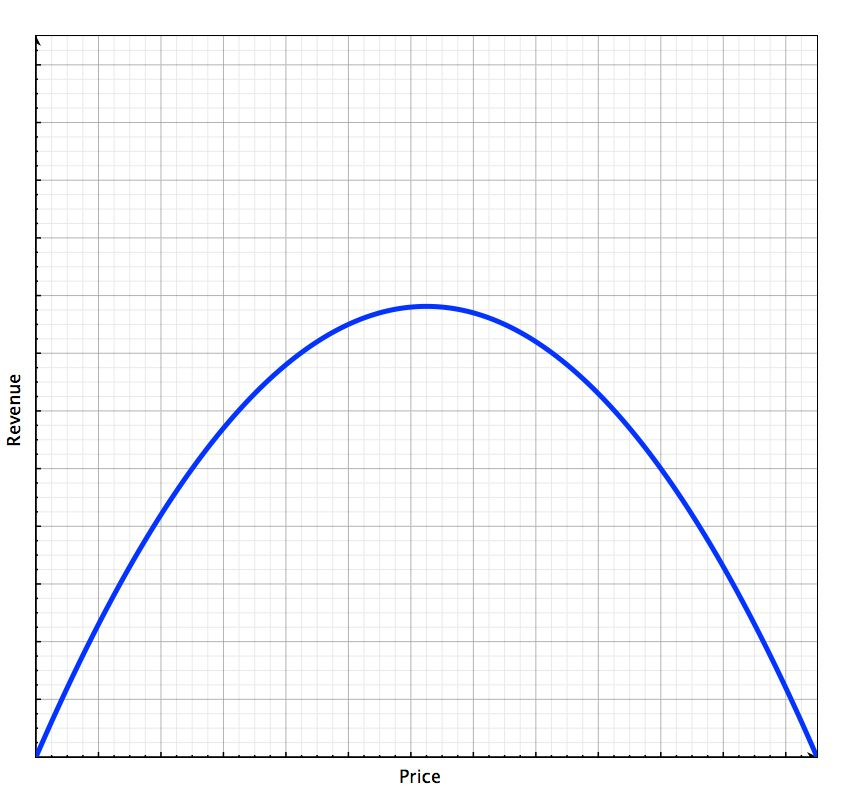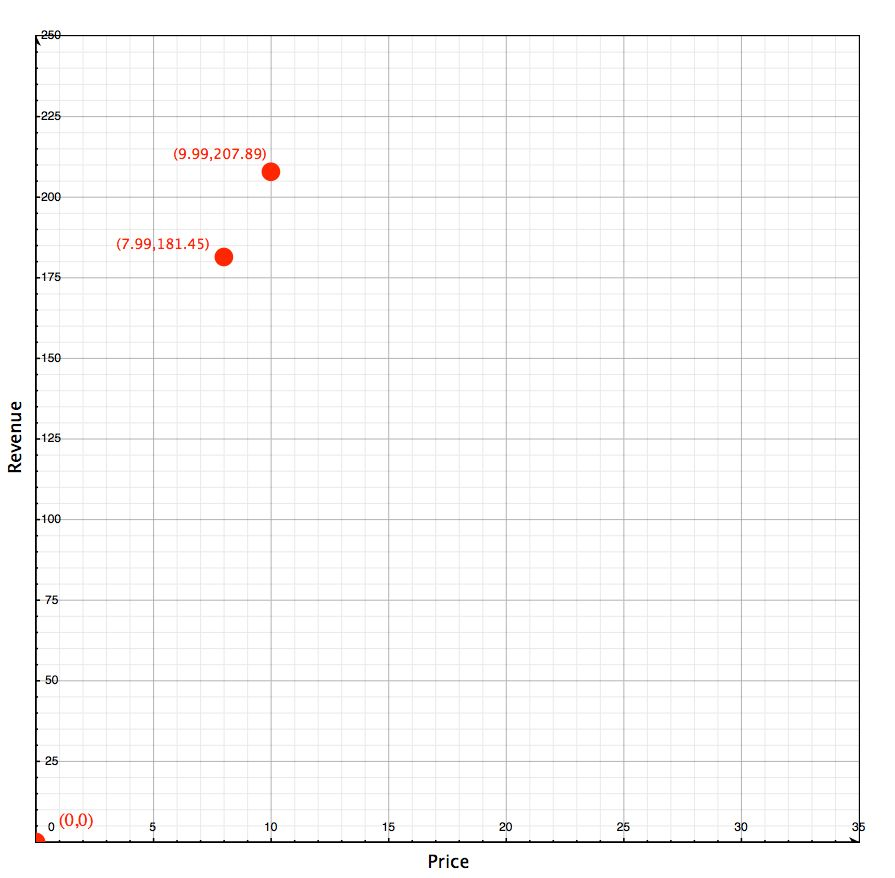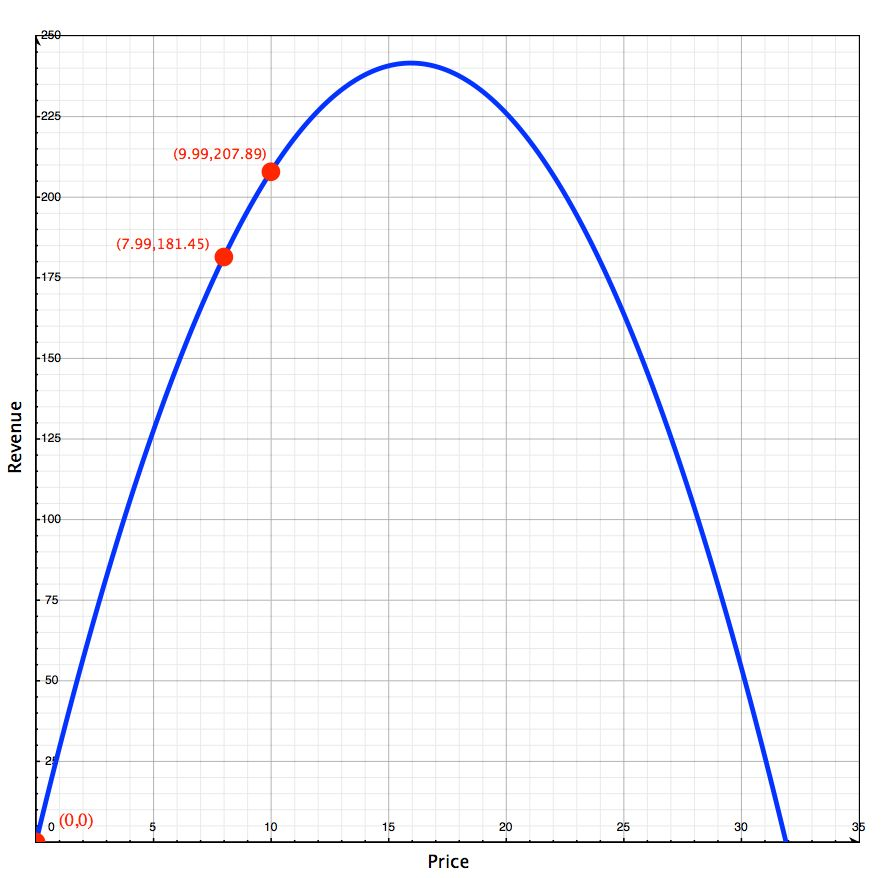## Optimizing the Price of Your App

###### Posted on October 4, 2013

There’s been a lot of talk recently about pricing apps on the App Store. Marco Arment thinks that mass market paid apps are dead. Joe Cieplinski, my co-host on Release Notes, thinks there’s still plenty of room for paid apps in the right markets. And Matt Gemmell thinks you should raise your price by a dollar, and then measure the effect it has. With all of this seemingly conflicting advice, what’s an app developer to do? We all want our apps to earn as much as possible, but what price point will achieve that goal? Two dollars? Five dollars? Ninety-nine cents? The fact is, most of us just guess. We pick a price that we think people will pay and we go with it. But what if there was another way? A way to make a reasonable estimate of the price point that would maximize the revenue for an app? A way that didn’t rely on gut feelings and best hopes, but rather economics and mathematics? Well, my friends, if we had that, we’d really be taking control of our software business.Why would a business owner want to be careful with the price he sets for his goods? Because price influences revenue, and revenue’s what we all want. It’s all the money that comes in the door. Ideally, in large quantities. Unfortunately, the relationship between price and revenue isn’t linear, as is apparent to anyone who thinks about it for more than a minute. If it were, we’d all just charge \$1000 per download and be App Store millionaires. Instead, as shown in the figure to the right, revenue starts to increase as we increase our price from free, but then we reach a point where revenue begins to decrease as we scare off potential customers with our higher prices. Our job as business owners, then, is to find the price at which revenue peaks so that we can earn the most from our investment in time and energy.

Finding that peak is the tricky bit, of course. Most developers aren’t trained in economics, and there’s not a lot of App Store-specific information available on the internet to guide us. One notable exception is a series of articles written by Michael Jurewitz, who is trained in economics. Jurewitz’s series on app pricing and the economics of the App Store (parts 1 2 3 4 5) has a lot of great information, and should be considered required reading for anyone who hopes to make a living in the App Store. My goal today, is to add to this existing work and describe a way that developers can calculate the price that optimizes revenue, rather than relying on experimentation. The calculation won’t be exact, but it should allow developers and business owners to find a price that’s close to optimum relatively quickly, and without having to rely on guesses.

Above, I wrote that a product’s revenue increases as its price increases up to a point, then revenue begins to decrease. Economists tell us that when you plot all of these price vs. revenue points, the resulting shape is a parabola. ((This assumes a linear demand curve, but more on that later.)) This is a key insight, because we know a lot about parabolas. For instance, every parabola has a quadratic function that describes it, of the form:

`y = ax2 + bx + c`

We also know, using our knowledge of algebra, that the peak (or vertex) of a parabola can be found where:

`x = -b / (2a)`

In our particular case, the independent variable is price (P), and the dependent variable is revenue (R), so if we replace x with P, and y with R, our equations become:

```R = aP2 + bP + c
P = -b / (2a)
```

So how is this useful? Well, if you know the equation of the parabola that fits your app’s price vs. revenue curve, you can use algebra to predict what price should result in the most revenue. There’s only one problem: You probably don’t know the equation of your particular parabola. Luckily, the equation can be found with just a little bit of price experimentation.

It turns out that you only need three points (P,R) to find the equation of a parabola, and you probably already have two of them. You know that if you give your app away for free, you’ll have zero revenue from sales. So you know that your parabola goes through the point (0,0). You also should be able to find your app’s average daily revenue at its current price by looking at your App Store statistics, or by using a product like AppViz or App Annie. That gives you another point. So all you have to do is alter your price once, allow revenue to come to a steady state over a month or so, and then find a third point.

At this point, an example is probably called for. Let’s imagine that Alice develops an app called “Homecalc,” which is a calculator app meant specifically for real estate agents. Homecalc provides value to its customers by making many calculations that are common to the real estate market easy and convenient. Alice has charged \$7.99 for Homecalc since its launch and she sees from her App Store reports that it makes an average of \$181.45 per day. A very profitable app, indeed. Good for her! But can she do better?To find out, Alice needs the equation of that parabola. She knows from her App Store reports that the parabola goes through the point (7.99,181.45). She also knows that the parabola goes through the point (0,0). But she needs one more data point. To get that last point, Alice decides to raise Homecalc’s price to \$9.99 and see what happens. After waiting a month or two, Alice looks at her reports and finds that Homecalc averaged \$207.89 per day in revenue after the price increase. That means that the parabola must also go through the point (9.99,207.89). That’s three points. Let’s find Alice’s equation.

I wrote earlier that the equation of an app’s price vs. revenue curve takes the form of:

`R = aP2 + bP + c`

Knowing that, Alice can take the three points that she found and plug them into the equation above. In this case, Alice has the points (0,0), (7.99,181.45), and (9.99,207.89). Plugging those values into our equation for the price vs. revenue curve, Alice gets three more equations:

```0 = a(0)2 + b(0) + c
181.45 = a(7.99)2 + b(7.99) + c
207.89 = a(9.99)2 + b(9.99) + c
```

which, when simplified, result in the equations:

```0a + 0b + c = 0
63.8401a + 7.99b + c = 181.45
99.80001a + 9.99b + c = 207.89
```

You will no doubt recognize that what Alice has created is a system of three equations in three variables. This system can be solved in any number of ways, but the easiest way is probably to allow technology to do the work. Using an online system of equations solver Alice found that the solution to this system (rounded to two decimal places) is:

```a = -0.95
b = 30.30
c = 0
```

When Alice plugs those values into our equation for the price vs. revenue curve:

`R = aP2 + bP + c`

she gets:

`R = -0.95P2 + 30.30P + 0`

which describes the price vs. revenue parabola that is unique to Homecalc!Alice graphed Homecalc’s price vs. revenue equation to get a better feel for where she should set her price next. Looking at the graph (shown to the right), it’s clear that Alice still has room to raise the price of Homecalc and increase its daily revenue. In fact, it looks from the graph that the optimum price for Homecalc is between \$15 and \$16. It also appears that by raising her price to that range, she can make another \$7 dollars per day. Of course, these numbers have been arrived at just by examining the graph. But if Alice wanted to actually calculate the optimum price for Homecalc, she could do that too.

To calculate the optimum price for Homecalc, Alice needs the values of a, b, and c that she calculated:

```a = -0.95
b = 30.30
c = 0
```

and the equation for optimum price that we established earlier:

`P = -b / (2a)`

Plugging a, and b into our equation, we find that

`P = -30.30 / [2 * (-0.95)]`

or, simplified,

`P = 15.95`

With this knowledge, it appears that Alice would be wise to set Homecalc’s price to \$15.99. If she does, our calculations show that she can expect an average daily revenue of \$241.60 per day. Compared to where she started, that’s an increase in revenue of about \$60 per day or almost \$22,000 per year! Will everyone see results like this? No way. These numbers were chosen, for the sake of illustration, to exaggerate the shape of the parabola. But I bet that most will see some improvement.